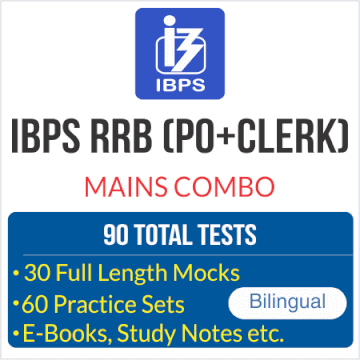Reasoning is a game of wits and presence of mind! Yes, it is true and it might seem as the greatest of the challenge after English Section’s surprises but yet this one can easily be dealt with. You just need correct practice and hardwire your brain to quickly make decisions of what to attempt and what to leave. And for same we are providing you questions of Reasoning Question and Answers. Solve these to Practice latest pattern reasoning question for bank exams.

Directions (1-5): In these questions, relationship between different elements is shown in the statements. These statements are followed by two conclusions. Mark answer-
(a) If only conclusion I follows.
(b) If only conclusion II follows.
(c) If either conclusion I or II follows.
(d) If neither conclusion I nor II follows.
(e) If both conclusions I and II follow.

Q1. . Statements: P ≥ Q > S, S > A < B > C
Conclusions: I. A ≥ C II. C = A

Q2. Statements: A > B > C ≥ D, C > P < Q
Conclusions: I. Q > A II. A ≥ Q

Q3. Statements: M ≥ P ≤ N, A ≥ O = N
Conclusions: I. M ≥ N II. A > P

Q4. Statements: A > B = C, D ≤ C, D < O ≤ M
Conclusions: I. A > D II. M > C

Q5. Statements: P <Q ≤ S, A < Y < S
Conclusions: I. P < S II. A < S

Direction (1-5):
S1.Ans.(d)
Sol.  I. I. A ≥ C (false)     II. C = A (false)

S2.Ans.(c)
Sol.  I. Q> A(false)         II.A ≥ Q (false)

S3.Ans.(d)
Sol.  I. M ≥ N (false)        II. A > P (false)

S4.Ans.(a)
Sol.  I. A > D (True)         II. M > C (false)

S5.Ans.(e)
Sol.  I. P < S (True)   II. A < S (True)

Directions (6-10): In each of the questions given below, a group of digits/letter is given followed by four combinations of symbols numbered (a), (b), (c) and (d). You have to find out which of the four combinations correctly represents the group of digits/letters based on the symbol codes and the conditions given below. If none of the four combinations represents the group of digits correctly, give (e) i.e. ‘None of these’ as your answer.
Condition for coding the group digits:
(i) If the 2nd and 5th element is vowel, then codes for the first and the last digits are to be interchanged.
(ii) If the first as well as the last element is consonant, then both are to be coded by the code for the last element.
(iii) If the first and last element is vowel, then both are to be coded as the code for 1st element.
(iv) If the 1st element is a digit and the last letter is consonant than both are to be coded as ‘=’.

Q6. ACE36E
(a) >9%#®>
(b) ∞9%<®∞
(c) >9%®#>
(d) >9#%®>
(e) None of these

Q7. NICKE3
(a) #∞¥9%?
(b) #∞¥9¥%?
(c) #∞9¥%?
(d) #∞9¥%#
(e) None of these

Q8.AIME3E
(a) >∞#?%>
(b) >∞≠%#>
(c) ≠∞?%%≠
(d) ≠∞%9≠
(e) None of these

Q9. 36RIM
(a) =®∞>#
(b) =®Å∞=
(c) =®∞ Å=
(d) =>∞ Å =
(e) None of these

Q10. KOMEX
(a) ∆\$%≠%∆
(b) ∆%\$≠%∆
(c) %∆\$≠%∆
(d) ∆\$%Å∆
(e) None of these

Directions (6-10):

S6.Ans.(a)
Sol. Condition III is applied. The Code for ICE36E is >9%#®>

S7.Ans.(c)
Sol. Condition I is applied. The Code for NICKE3 is #∞9¥%?

S8.Ans.(b)
Sol. Condition III is applied. The Code for AINE3E is >∞≠%#>

S9.Ans.(b)
Sol. Condition IV is applied. The Code for 36AIM is =®Å∞=

S10.Ans.(e)
Sol. Condition II is applied. The Code for KOMEX is ∆\$≠%∆

Directions (11-15): Study the following information carefully and answer the questions given below:
There are eight persons A, B, C, D, E, F, G and H are sitting around a square table in such a way that four of them sit at four corners and are facing center, while those who sits in the middle of the side of the square are facing outside the centre. They all likes different color Blue, Black, Brown, Red, Yellow, Pink, Purple and Green but not necessarily in the same order.
D likes Blue color and sits third to the right of E, who doesn’t like either pink or Red. H sits at the corner. The one who likes Red color sits opposite the one who likes Green color. B does not like Red color. B sits 3rd to the right of C who likes Black color. G likes Brown color and faces outside the centre. The one who likes Blue color sits immediate left of one who likes Brown color. The one who likes yellow color sits 2nd to the right of one who likes Purple color. H likes Red color and sits 2nd to the left of A.

Q11. Who among the following likes Pink color?
(a) A
(b) E
(c) F
(d) C
(e) D

Q12. B likes which of the following color?
(a) Red
(b) Green
(c) Yellow
(d) Red
(e) Pink

Q13. In the given question some follows a pattern find the one which is not following the pattern?
(a) A and D
(b) F and C
(c) E and G
(d) D and E
(e) H and B

Q14. If A is related to Blue, and in the same way F is related to Black then following the same pattern D is related to?
(a) Blue
(b) Green
(c) Pink
(d) Brown
(e) Red

Q15. Which of the following condition is/are is correct about B?
(a) B faces outside the centre
(b) B likes red color
(c) B sits third to the right of E
(d) All are correct
(e) B sits 2nd to the right of A

Directions (11-15):

S11. Ans.(a)
Sol.

S12. Ans.(b)
Sol.

S13. Ans.(d)
Sol.

S14. Ans.(c)
Sol.

S15. Ans.(e)
Sol.

You May also like to Read: